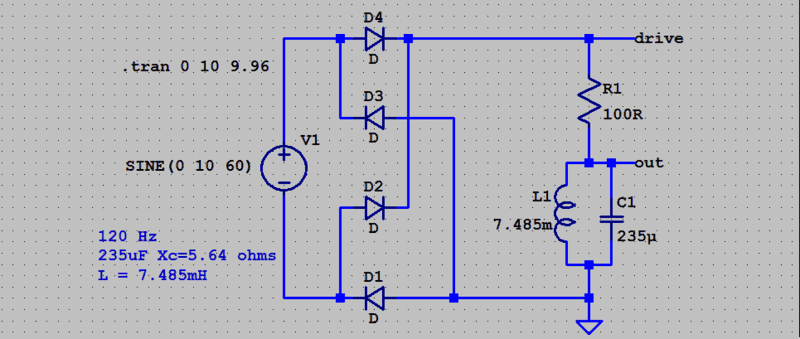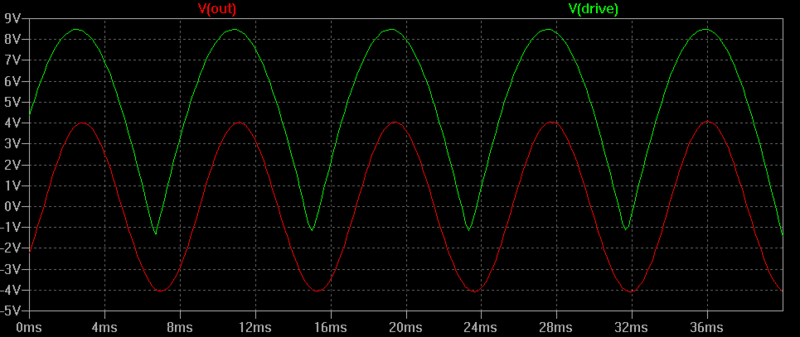# Pulsating DC supply to LC circuit

• electricalguy
If you only require adding a series resistance of 186.9 ohms to the primary winding of the transformer, you can do so by adding a resistor in series with the primary winding.f

#### electricalguy

Gold Member
I have a very basic question for the professionals here, but I am having a small issue with my parallel LC circuit. I have an inductor that is 6.425mH, a capacitor that is 217uF (it is two 470uF aluminum electrolytic caps connected in series to make one non-polar 217uF cap). I am supplying the circuit with 120 Hz pulsating DC voltage of roughly 6.3 volts and 2.8 amps. The supply comes from a 60hz AC supply from a small transformer through a bridge rectifier. The problem being I am not getting a resonance effect at all. Based on my online calculations I should have a circuit impedance of roughly 17 to 20 ohms. But it is staying around 2 to 3 ohms. That tells me the circuit isn't resonating. How can I get this circuit to resonate. I know the values don't exactly match 120hz, but there should be some resonance effect.

Can you post the full schematic please? I can guess or assume, but you know what happens when we do that...Based on my online calculations I should have a circuit impedance of roughly 17 to 20 ohms.
show that calculation?

EDIT Ignore what follows - i thought he said Series resonant . Please Pardon Me !
Series resonance lowers impedance.
What do you calculate for XL and XC ?

How can I get this circuit to resonate.
How do you know it's not resonating?
Quick check -
Measure AC(not DC) voltage across the cap, write it here________
and the inductor, write it here ____________
and the combination of the two(rectifier output ?) , write it here__________

At or near resonance the sum will be less than either of its parts. Can you explain why?

Last edited:
It's also worth remembering that standard electrolytics have tolerances of +50%/-20%.
The equivalent series resistance of electrolytics is also significant and will make your tuned cct very low Q.

•CWatters and Tom.G
I have a very basic question for the professionals here, but I am having a small issue with my parallel LC circuit. I have an inductor that is 6.425mH, a capacitor that is 217uF (it is two 470uF aluminum electrolytic caps connected in series to make one non-polar 217uF cap). I am supplying the circuit with 120 Hz pulsating DC voltage of roughly 6.3 volts and 2.8 amps. The supply comes from a 60hz AC supply from a small transformer through a bridge rectifier. The problem being I am not getting a resonance effect at all. Based on my online calculations I should have a circuit impedance of roughly 17 to 20 ohms. But it is staying around 2 to 3 ohms. That tells me the circuit isn't resonating. How can I get this circuit to resonate. I know the values don't exactly match 120hz, but there should be some resonance effect.
The parallel LC circuit is also in parallel with the power supply. As the latter has low resistance, the total parallel resistance is also very small. The Q of the circuit is given by Rp/X and so if Rp is small, Q is small and the resonance action is reduced or destroyed.
Try placing a high resistance in series with the supply. Now you will find a voltage peak across L or C as frequency is altered

•Tom.G and jim hardy
Thank you everyone for your assistance. With the suggestion to add a high resistance in series with the supply, do you mean to add that resistance in series with the primary winding of the transformer? The primary winding has a resistance of 25.5 ohms and a reactance of 168.96 ohms. Correct me if I'm wrong but that suggest a Q of 0.150923295. If I wanted a Q of 1.24715, I would require a resistance of 212.4088196 ohms. Which would be adding an extra 186.9088196 ohms. The secondary winding of the transformer which is connected to the parallel LC circuit has a resistance of 2.4 ohms and a reactance of 5.120 ohms. That equals a Q of 0.46875. So if I were to get a Q of 1.247154472 I would require a series resistance on the secondary winding of 6.38543 ohms. That would equal an addition of 3.9854 ohms. So adding to my question above, do I only require adding a series resistance of 186.9 ohms to the primary winding of the transformer?

The primary winding has a resistance of 25.5 ohms and a reactance of 168.96 ohms. Correct me if I'm wrong but that suggest a Q of 0.150923295.

Q = X/R = ~ 25.5/169 = 1/(your number)

old jim

Thank you everyone for your assistance. With the suggestion to add a high resistance in series with the supply, do you mean to add that resistance in series with the primary winding of the transformer? The primary winding has a resistance of 25.5 ohms and a reactance of 168.96 ohms. Correct me if I'm wrong but that suggest a Q of 0.150923295. If I wanted a Q of 1.24715, I would require a resistance of 212.4088196 ohms. Which would be adding an extra 186.9088196 ohms. The secondary winding of the transformer which is connected to the parallel LC circuit has a resistance of 2.4 ohms and a reactance of 5.120 ohms. That equals a Q of 0.46875. So if I were to get a Q of 1.247154472 I would require a series resistance on the secondary winding of 6.38543 ohms. That would equal an addition of 3.9854 ohms. So adding to my question above, do I only require adding a series resistance of 186.9 ohms to the primary winding of the transformer?
As we are not studying transformers as part of the exercise, I had in mind to place a high value resistor, such as 1000 Ohms, in series with the secondary. This will allow the LC circuit to have almost its maximum Q. Another comment is that if the Q is below 0.5, the circuit is not really resonant. If a DC impulse is applied to the circuit, it does not reverse the polarity and it does not create a wave.

Here is a simulation with slightly different values for L and for C.Notice the slight phase shift between drive and LC voltage due to not being accurately tuned.